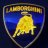# Help with extending Pivots to the right indefinitely

H

#### hedgestan

##### New member
VIP
Hi, I'm trying to figure out how to extend the Woodies Pivot from the previous time period to the right. This is the standard Woodies Pivot Script.

This study is using the yearly timeframe to plot pivots.Code:
``````#
# TD Ameritrade IP Company, Inc. (c) 2009-2020
#

input timeFrame = {default DAY, "2 DAYS", "3 DAYS", "4 DAYS", WEEK, MONTH, "OPT EXP", QUARTER, YEAR};
input showOnlyToday = no;

def highByPeriod = high(period = timeFrame);
def lowByPeriod = low(period = timeFrame);
def openByPeriod = open(period = timeFrame);
def closeByPeriod = close(period = timeFrame)[-1];

plot R3;
plot R2;
plot R1;
plot PP;
plot S1;
plot S2;
plot S3;

if showOnlyToday and !IsNaN(closeByPeriod)
then {
R1 = Double.NaN;
R2 = Double.NaN;
R3 = Double.NaN;
PP = Double.NaN;
S1 = Double.NaN;
S2 = Double.NaN;
S3 = Double.NaN;
} else {
PP = (highByPeriod + lowByPeriod + 2 * openByPeriod) / 4;
R1 = 2 * PP - lowByPeriod;
S1 = 2 * PP - highByPeriod;
R2 = PP + R1 - S1;
S2 = PP + S1 - R1;
R3 = R2 + R1 - PP;
S3 = S2 + S1 - PP;
}

PP.SetDefaultColor(GetColor(0));
R1.SetDefaultColor(GetColor(5));
R2.SetDefaultColor(GetColor(5));
R3.SetDefaultColor(GetColor(5));
S1.SetDefaultColor(GetColor(6));
S2.SetDefaultColor(GetColor(6));
S3.SetDefaultColor(GetColor(6));

PP.SetStyle(Curve.SHORT_DASH);
R1.SetStyle(Curve.SHORT_DASH);
R2.SetStyle(Curve.SHORT_DASH);
R3.SetStyle(Curve.SHORT_DASH);
S1.SetStyle(Curve.SHORT_DASH);
S2.SetStyle(Curve.SHORT_DASH);
S3.SetStyle(Curve.SHORT_DASH);

def paintingStrategy = if timeframe == timeframe.WEEK then PaintingStrategy.LINE_VS_TRIANGLES else if timeFrame == timeFrame.MONTH or timeFrame == timeFrame."OPT EXP" or timeFrame == timeFrame.QUARTER or timeFrame == timeFrame.YEAR then PaintingStrategy.LINE_VS_SQUARES else PaintingStrategy.LINE_VS_POINTS;

PP.SetPaintingStrategy(paintingStrategy);
R1.SetPaintingStrategy(paintingStrategy);
R2.SetPaintingStrategy(paintingStrategy);
R3.SetPaintingStrategy(paintingStrategy);
S1.SetPaintingStrategy(paintingStrategy);
S2.SetPaintingStrategy(paintingStrategy);
S3.SetPaintingStrategy(paintingStrategy);``````Help with a "def" statement Questions 4